# Math in Focus Grade 5 Chapter 5 Practice 1 Answer Key Number Patterns and Relationships

Go through the Math in Focus Grade 5 Workbook Answer Key Chapter 5 Practice 1 Number Patterns and Relationships to finish your assignments.

## Math in Focus Grade 5 Chapter 4 Practice 1 Answer Key Number Patterns and Relationships

Write the next three terms of each number pattern. Then describe the rule for each pattern.

Question 1.
3, 6, 9, 12, __, ___, ____
Rule: ____
3, 6, 9, 12, 15, 18, 21
Rule: Multiples of 3.
Explanation:
The next three terms of a given number pattern 3, 6, 9, 12 is 15, 18, 21.

Question 2.
4,000,000, 400,000, 40,000, 4,000, ___, ___,
____,
Rule: ____
4,000,000, 400,000, 40,000, 4,000, 4,00, 40, 4.

Question 3.
3, 6, 12, 24, __, ___, ____
Rule: _____
3, 6, 12, 24, 48, 96, 192

Question 4.
50, 49, 47, 44, 40, __, ___, ____
Rule: _________
50, 49, 47, 44, 40, 35, 29, 22

Complete the tables.

Question 5.
a.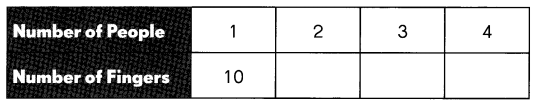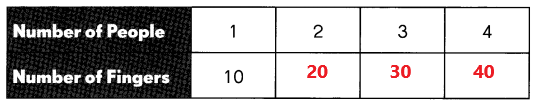b.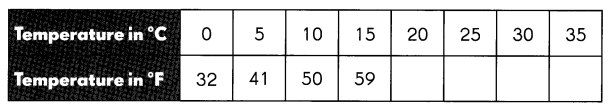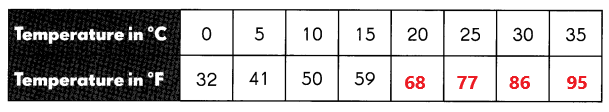Complete the table and answer the questions.

Question 6.
The table shows the relationship between temperatures measured in degrees Celsius (°C) and degrees Fahrenheit (°F).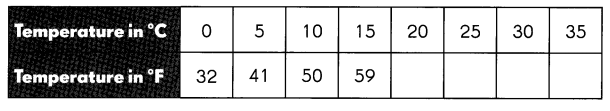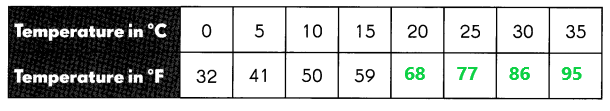a. If the temperature is 100°F outside, is this temperature greater than or less than 40°C? Explain.

b. Recently meteorologists added a new color to their weather maps for temperatures of 1 22°F or greater. What temperature is this in degrees Celcius?

Complete the tables and answer the questions.

Question 7.
A set of 20 plastic cups costs $2.50.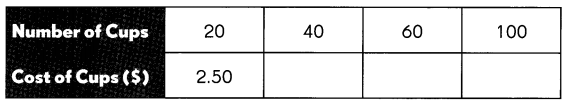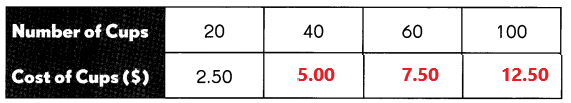a. What is the cost of 60 cups? Answer:$2.50 + $2.50 +$2.50 = $7.50 Explanation: The cost of 60 cups is$7.50.

b. What is the cost of 8 sets of cups?
20 cups x 8 = 160 cups
$2.50 x 8 =$20
The cost of 160 cups is $20. Explanation: A set of 20 plastic cups costs$2.50. Multiply $2.50 with 8 the product is$20. The cost of 8 sets of cups is $20. c. How many cups can you get for$17.50?
20 cups x 7 = 140 cups
$2.50 x 7 =$17.50.
Explanation:
A set of 20 plastic cups costs $2.50. Multiply$2.50 with 7 the product is $17.50. We can get 140 cups for$17.50.

Question 8.
A van travels at a speed of 60 kilometers per hour.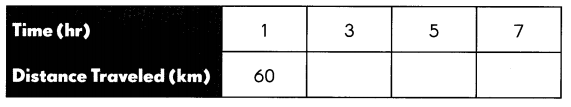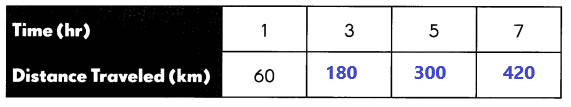a. How far does the van travel in 6 hours?
1 hour = 60 km
6 hours = ?
60 x 6 = 360km
Explanation:
A van travels at a speed of 60 kilometers per hour. Multiply 6 hours with 60 kilometers the product is 260 kilometers. The van travels 360 kilometers in 6 hours.

b. How long does the van take to travel 180 kilometers?
1 hour = 60 km
? = 180 km
180km x 1 hour = ? 60 km
180/ 60 = 3hours
Explanation:
A van travels at a speed of 60 kilometers per hour. Divide 180 by 60 the result is 3. The van takes 3 hours to travel 180 kilometers.

c. How long does the van take to travel 180 kilometers?
Alex borrowed $155 from his parents to buy an electronic notepad. He repays some of the loan each week. The table shows how much he still owes after he repays the same amount each week.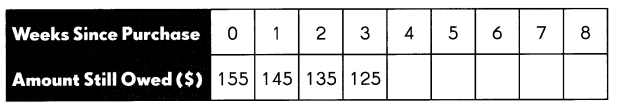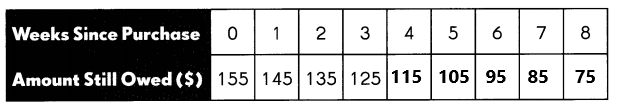a. How much of his loan is Alex repaying each week? Answer: Alex repaying each week$10 of his loan.
At week 10 Alex left to repay $55. c. Will it take Alex 15 weeks to repay the loan? Explain. Answer: Alex takes 16 weeks to repay the loan. Every week he repaying$10. At 15 week Alex left to repay \$5.